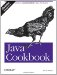# Recipe 5.2 Storing a Larger Number in a Smaller Number

#### Problem

You have a number of a larger type and you want to store it in a variable of a smaller type.

#### Solution

Cast the number to the smaller type. (A cast is a type listed in parentheses before a value that causes the value to be treated as though it were of the listed type.)

For example, to cast a long to an int, you need a cast. To cast a double to a float, you also need a cast.

#### Discussion

This causes newcomers some grief, as the default type for a number with a decimal point is double, not float. So code like:

`float f = 3.0;`

won't even compile! It's as if you had written:

`double tmp = 3.0;  float f = tmp;`

You can fix it by making f a double, by making the 3.0 a float, by putting in a cast, or by assigning an integer value of 3:

`double f = 3.0;  float f = 3.0f;  float f = 3f;  float f = (float)3.0;  float f = 3;`

The same applies when storing an int into a short, char, or byte:

`// CastNeeded.java  public static void main(String argv[]) {      int i;      double j = 2.75;      i = j;            // EXPECT COMPILE ERROR      i = (int)j;        // with cast; i gets 2      System.out.println("i =" + i);      byte b;      b = i;            // EXPECT COMPILE ERROR      b = (byte)i;    // with cast, i gets 2      System.out.println("b =" + b);  }`

The lines marked EXPECT COMPILE ERROR do not compile unless either commented out or changed to be correct. The lines marked "with cast" show the correct forms.Java Cookbook, Second Edition
ISBN: 0596007019
EAN: 2147483647
Year: 2003
Pages: 409
Authors: Ian F Darwin

Similar book on Amazon